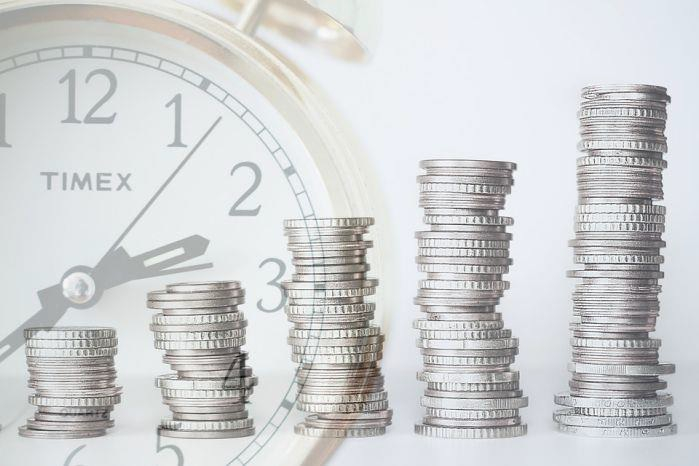# What is Definition, Formula & Example of Key Rate Duration?

## What is key rate duration?

The key rate duration of a debt security or a debt instrument portfolio, often bonds, examines how the value of a debt security or portfolio varies at a certain maturity point throughout the whole yield curve. With respect to other maturities, the key rate duration is used to determine how sensitive a debt security's price is to a one percent change in yield for a certain maturity while all other maturities are held constant.## Key Points Briefly

• Using the key rate duration, you can determine how much a bond's price changes when the yield on the bond changes by one percentage point (100 basis points) throughout its entire term.

• As the yield curve moves in a parallel direction, you may use effective duration to estimate portfolio value changes. However, when there is a non-parallel shift of yield-curve, you must utilize key rate duration to estimate portfolio value adjustments.

• Length measures inform you of the price risk associated with owning fixed income instruments in the event of a change in the interest rate environment.

## What is Key Rate Duration Formula?

Here, we will look at key rate duration formula and understand it better with an example.

$$Key\:rate\:duration=\frac{P_{-}-P_{+}}{2*0.01*P_{0}}$$

Where −

P- − Price of bond after 1% decreases in profit.

P+ − Price of bond after 1% increase in profit.

P0 − Original price of the bond

## Key Rate Duration - Example

Let us take the example of the asset, whose original price is 1,575 and there was a fall in in its value to \$1,250 due when there was 1 percent rise in its bond maturity. However, there was a price increase to 1,750 when that bond maturity value dropped.

Using the above formula, and the numbers from the example, let us calculate the key rate definition for our asset.

The key rate duration will be (1750 – 1250) / (2 * 0.01 * 1575) = 15.87

## The Importance of the Key Rate's Time Duration

The predicted change in value resulting from a change in the yield on a bond or bond portfolio with a given maturity is represented by the key rate duration. It is presumptively assumed that the yields for all other maturities would remain constant.

Assuming that an investor's interest rate predictions prove to be correct over a given time period, they can use the key rate duration metric to determine which bond maturities are most likely to provide the most profitable investment returns (assumed that the investor's interest rate predictions prove to be correct). As a result, the statistic may be used to compare different potential fixed-income investments.

When an investor owns a debt security, determining key rate duration may be beneficial in a number of other situations as well. They may be interested in estimating the change in the value of the bond they own as a result of different basis point fluctuations. This may assist customers in determining the possibility that their bond may be called in for early redemption by the issuer of the bond.

Updated on: 02-Jul-2021

324 Views